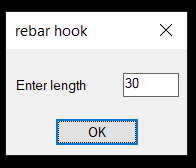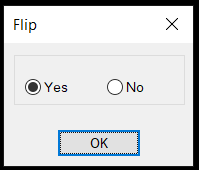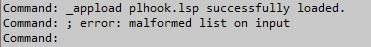# REQ : lisp to draw polyline rebar end hook side indecator

## Recommended Postshello .

i am new at this forum

can you help me to write lisp  .

thanks for all worthily efforts

abdulellah.alattab@gmail.com

Edited by Abdulellah

##### Share on other sites10 minutes ago, Abdulellah said:

hello .

i am new at this forum

can you help me to write lisp  .

thanks for all worthily efforts

-select polyline
-ask hook side at start point and it is length and angle (preferd 45 degree )
-ask hook side at end point and it is length and angle (preferd 45 degree )

abdulellah.alattab@gmail.com

##### Share on other sitesThis is a good time to start to learning lisp, without getting to smart about the task you want a extra line either left or right of a pline end.

This would be done using the endpoint and startpoint of a pline, then using the polar command working out a new point adding the line then to the pline.

So a start get the pline vertice points this allows you to work out the pline end segments angles. using polar command draw the new line +45 to the angle ask is it correct direction if not erase and use -45 to the angle.

Ok so some code

```(setq plent (entsel "Pick pline"))
(if plent (setq co-ord (mapcar 'cdr (vl-remove-if-not '(lambda (x) (= (car x) 10)) (entget (car plent))))))
; ((156.0 78.0) (307.957959107735 78.0) (307.957959107735 203.997487733392)) ; this is a L pline 3 points
(setq pt1 (nth 0 co-ord)) ; 1st point
(setq pt2 (nth 1 co-ord)) ; second point
(setq x (- (length co-ord) 1)) ; how many points- 1 as 1st point is 0
(setq pt3 (nth (- x 1) co-ord)) ; second last point
(setq pt4 (nth x  co-ord)) ; last point

(setq ang1 (angle pt2 pt1)) ; angle ofthe pline
(setq ang2 (angle pt3 pt4))

Pt5 using polar command
then line pt1 pt5
if wrong direction use mirror command note angle must be in radians so use (* 0.75 pi) for 135 degrees.```

Please to others have to start at some point.

Complete code to do what you want it is short.

Edited by BIGAL
•1

##### Share on other sitesthanks BIGAL , i dont have any idea about auto lisp programming , i hope to help me if you can , please

##### Share on other sitesok . we can reduce tasks to one task as this order

- enter lisp command in prompt line like hook ( say hok )

- select point which  you want to add hook regardless if at poly line end  or not

-

##### Share on other sitesYes there are more things to do with this code but I would like this to be a learning example, getint is the next step or see image below.

`see last post`Edited by BIGAL
•1

##### Share on other sites21 hours ago, BIGAL said:

Yes there are more things to do with this code but I would like this to be a learning example, getint is the next step or see image below.

```
(defun c:plhook ( / plent pt1 pt2 pt3 x pt5 add ang1 ang2)
(setq plent (entsel "Pick pline"))
(if plent (setq co-ord (mapcar 'cdr (vl-remove-if-not '(lambda (x) (= (car x) 10)) (entget (car plent)))))

(setq pt1 (nth 0 co-ord)) ; 1st point
(setq pt2 (nth 1 co-ord) ; second point
(setq x (- (length co-ord) 1)) ; how many points- 1 as 1st point is 0
(setq pt3 (nth (- x 1) co-ord)) ; second last point
(setq pt4 (nth x  co-ord)) ; last point

(setq ang1 (angle pt2 pt1)) ; angle ofthe pline
(setq ang2 (angle pt3 pt4))

(setq pt5 (polar pt1 (+ (* 0.75 pi)) add)) ; 135 degrees
(command "line" pt1 pt5 "") ; new line
(setq ans (getstring "is line ok Y or N")) ; is it in correct direction
(if (= "Y" (strcase ans)) ; y or Y
(princ "ok")
(command "mirror" "Last" "" pt1 pt2 "Y") ; flip line
)
(setq pt5 (polar pt4 (+ (* 0.75 pi)) add)) ; other end
(command "line" pt4 pt5 "")
(setq ans (getstring "is line ok Y or N"))
(if (= "Y" (strcase ans))
(princ "ok")
(command "mirror" "Last" "" pt1 pt2 "Y")
)
)
)
(c:plhook)```Edited by Abdulellah

##### Share on other sites##### Share on other sitesRe doing was typo in code.

```(defun c:plhook ( / plent pt1 pt2 pt3 x pt5 add ang1 ang2)
(while (setq plent (entsel "Pick pline Enter to exit"))
(if plent (setq co-ord (mapcar 'cdr (vl-remove-if-not '(lambda (x) (= (car x) 10)) (entget (car plent))))))
(setq pt1 (nth 0 co-ord)) ; 1st point
(setq pt2 (nth 1 co-ord)) ; second point
(setq x (- (length co-ord) 1)) ; how many points- 1 as 1st point is 0
(setq pt3 (nth (- x 1) co-ord)) ; second last point
(setq pt4 (nth x  co-ord)) ; last point
(setq ang1 (angle pt2 pt1)) ; angle ofthe pline
(setq ang2 (angle pt3 pt4))
(setq pt5 (polar pt1 (+ (* 0.25 pi) ang1) add)) ; 135 degrees
(command "line" pt1 pt5 "") ; new line
(setq ans (getstring "is line ok Y or N")) ; is it in correct direction
(if (= "Y" (strcase ans)) ; y or Y
(princ "ok")
(command "mirror" "Last" "" pt1 pt2 "Y") ; flip line
)
(setq pt5 (polar pt4 (+ (* 0.25 pi) ang2) add)) ; other end
(command "line" pt4 pt5 "")
(setq ans (getstring "is line ok Y or N"))
(if (= "Y" (strcase ans))
(princ "ok")
(command "mirror" "Last" "" pt4 pt3 "Y")
)
)
)
(c:plhook)```

Edited by BIGAL
•1

##### Share on other sitesOn 11/28/2019 at 6:23 AM, BIGAL said:

Re doing was typo in code.

```
(defun c:plhook ( / plent pt1 pt2 pt3 x pt5 add ang1 ang2)
(while (setq plent (entsel "Pick pline Enter to exit"))
(if plent (setq co-ord (mapcar 'cdr (vl-remove-if-not '(lambda (x) (= (car x) 10)) (entget (car plent))))))
(setq pt1 (nth 0 co-ord)) ; 1st point
(setq pt2 (nth 1 co-ord)) ; second point
(setq x (- (length co-ord) 1)) ; how many points- 1 as 1st point is 0
(setq pt3 (nth (- x 1) co-ord)) ; second last point
(setq pt4 (nth x  co-ord)) ; last point
(setq ang1 (angle pt2 pt1)) ; angle ofthe pline
(setq ang2 (angle pt3 pt4))
(setq pt5 (polar pt1 (+ (* 0.25 pi) ang1) add)) ; 135 degrees
(command "line" pt1 pt5 "") ; new line
(setq ans (getstring "is line ok Y or N")) ; is it in correct direction
(if (= "Y" (strcase ans)) ; y or Y
(princ "ok")
(command "mirror" "Last" "" pt1 pt2 "Y") ; flip line
)
(setq pt5 (polar pt4 (+ (* 0.25 pi) ang2) add)) ; other end
(command "line" pt4 pt5 "")
(setq ans (getstring "is line ok Y or N"))
(if (= "Y" (strcase ans))
(princ "ok")
(command "mirror" "Last" "" pt4 pt3 "Y")
)
)
)
(c:plhook)```

greate job herro , very usefull lisp , one more  thing for maximum benifit , can you do that with *. dcl window at right select hook side at start point , at left select hook side at end point .

after add hooks , can join both hooks to be with polyline as one object .

then select new connected polyline and fillet all poly segment together with predefined redius.

i am have confident to do that .

##### Share on other sitesMulti GETVALS.lspTry this

```(defun c:plhook ( / plent pt1 pt2 pt3 x pt5 add ang1 ang2)
(if (= but nil)(setq but 1))
(while (setq plent (entsel "Pick pline Enter to exit"))
(if (/= plent nil)
(progn
(setq co-ord (mapcar 'cdr (vl-remove-if-not '(lambda (x) (= (car x) 10)) (entget (car plent)))))
(setq pt1 (nth 0 co-ord)) ; 1st point
(setq pt2 (nth 1 co-ord)) ; second point
(setq x (- (length co-ord) 1)) ; how many points- 1 as 1st point is 0
(setq pt3 (nth (- x 1) co-ord)) ; second last point
(setq pt4 (nth x  co-ord)) ; last point
(setq ang1 (angle pt2 pt1)) ; angle ofthe pline
(setq ang2 (angle pt3 pt4))
(setq ans (AH:getvalsm (list "Enter length" "Hook length " 5 4 "30" "Radius" 5 4 "25" )))
(setq add (atof (nth 0 ans)))
(setq rad (atof (nth 1 ans)))
(setq pt5 (polar pt1 (+ (* 0.25 pi) ang1) add)) ; 135 degrees
(command "line" pt1 pt5 "") ; new line
(setq ans (ah:butts but "V" '("Flip  " "Yes" "No")))
(if (= "No"  ans)
(princ "ok")
(command "mirror" "Last" "" pt4 pt3 "Y")
)
(command "pedit" (car plent) "join" (entlast) "" "")
(setq pt5 (polar pt4 (+ (* 0.25 pi) ang2) add)) ; other end
(command "line" pt4 pt5 "")
(setq ans (ah:butts but "V" '("Flip  " "Yes" "No")))
(if (= "No"  ans)
(princ "ok")
(command "mirror" "Last" "" pt4 pt3 "Y")
)
(command "pedit" (car plent) "join" (entlast) "" "")
(command "fillet" "Polyline" "r" rad  (car plent))
)
)
)
)
(c:plhook)```

Edited by BIGAL
•1

##### Share on other sitesJust now, BIGAL said:

Try this

•1

##### Share on other sitesVery embarrassing, for your extra generosity, really you are a wonderful human

On 11/28/2019 at 6:23 AM, BIGAL said:

Re doing was typo in code.

```
(defun c:plhook ( / plent pt1 pt2 pt3 x pt5 add ang1 ang2)
(while (setq plent (entsel "Pick pline Enter to exit"))
(if plent (setq co-ord (mapcar 'cdr (vl-remove-if-not '(lambda (x) (= (car x) 10)) (entget (car plent))))))
(setq pt1 (nth 0 co-ord)) ; 1st point
(setq pt2 (nth 1 co-ord)) ; second point
(setq x (- (length co-ord) 1)) ; how many points- 1 as 1st point is 0
(setq pt3 (nth (- x 1) co-ord)) ; second last point
(setq pt4 (nth x  co-ord)) ; last point
(setq ang1 (angle pt2 pt1)) ; angle ofthe pline
(setq ang2 (angle pt3 pt4))
(setq pt5 (polar pt1 (+ (* 0.25 pi) ang1) add)) ; 135 degrees
(command "line" pt1 pt5 "") ; new line
(setq ans (getstring "is line ok Y or N")) ; is it in correct direction
(if (= "Y" (strcase ans)) ; y or Y
(princ "ok")
(command "mirror" "Last" "" pt1 pt2 "Y") ; flip line
)
(setq pt5 (polar pt4 (+ (* 0.25 pi) ang2) add)) ; other end
(command "line" pt4 pt5 "")
(setq ans (getstring "is line ok Y or N"))
(if (= "Y" (strcase ans))
(princ "ok")
(command "mirror" "Last" "" pt4 pt3 "Y")
)
)
)
(c:plhook)```

##### Share on other sitesSorry, if you can explain how to run attachments in the last reply, you only  can help me .

##### Share on other sitesYou need to save the two files in a Autocad supported path or edit the "Load path.

•1

##### Share on other sitesOn 11/28/2019 at 6:23 AM, BIGAL said:

Re doing was typo in code.

```
(defun c:plhook ( / plent pt1 pt2 pt3 x pt5 add ang1 ang2)
(while (setq plent (entsel "Pick pline Enter to exit"))
(if plent (setq co-ord (mapcar 'cdr (vl-remove-if-not '(lambda (x) (= (car x) 10)) (entget (car plent))))))
(setq pt1 (nth 0 co-ord)) ; 1st point
(setq pt2 (nth 1 co-ord)) ; second point
(setq x (- (length co-ord) 1)) ; how many points- 1 as 1st point is 0
(setq pt3 (nth (- x 1) co-ord)) ; second last point
(setq pt4 (nth x  co-ord)) ; last point
(setq ang1 (angle pt2 pt1)) ; angle ofthe pline
(setq ang2 (angle pt3 pt4))
(setq pt5 (polar pt1 (+ (* 0.25 pi) ang1) add)) ; 135 degrees
(command "line" pt1 pt5 "") ; new line
(setq ans (getstring "is line ok Y or N")) ; is it in correct direction
(if (= "Y" (strcase ans)) ; y or Y
(princ "ok")
(command "mirror" "Last" "" pt1 pt2 "Y") ; flip line
)
(setq pt5 (polar pt4 (+ (* 0.25 pi) ang2) add)) ; other end
(command "line" pt4 pt5 "")
(setq ans (getstring "is line ok Y or N"))
(if (= "Y" (strcase ans))
(princ "ok")
(command "mirror" "Last" "" pt4 pt3 "Y")
)
)
)
(c:plhook)```

mr.begal , some thing error  with this code , try to long multi segment poly , measure  angle , delete end lines , try it agine  please , belive me some thing error happen , can you refine it

##### Share on other sitesCould you upload a file with the pline before and after

##### Share on other sitesThis is latest has offset radius and angle pick pline anywhere.

```(defun c:plhook ( / plent pt1 pt2 pt3 pt4 ang3 pt5 add ang1 ang2 ang3)
(if (= but nil)(setq but 1))
(while (setq plent (entsel "Pick pline Enter to exit"))
(setq co-ord (mapcar 'cdr (vl-remove-if-not '(lambda (x) (= (car x) 10)) (entget (car plent)))))
(setq pt1 (nth 0 co-ord)) ; 1st point
(setq pt2 (nth 1 co-ord)) ; second point
(setq x (- (length co-ord) 1)) ; how many points- 1 as 1st point is 0
(setq pt3 (nth (- x 1) co-ord)) ; second last point
(setq pt4 (nth x  co-ord)) ; last point
(setq ang1 (angle pt2 pt1)) ; angle ofthe pline
(setq ang2 (angle pt3 pt4))
(setq ans (AH:getvalsm (list "Enter length" "Hook length " 5 4 "30" "Radius" 5 4 "25" "angle " 5 4 "45")))
(setq add (atof (nth 0 ans)))
(setq rad (atof (nth 1 ans)))
(setq ang3 (* pi (/ (atof (nth 2 ans)) 180.0)))

(setq pt5 (polar pt1 (+ ang3 ang1) add)) ; 135 degrees
(command "line" pt1 pt5 "") ; new line
(setq ans (ah:butts but "V" '("Flip  " "Yes" "No")))
(if (= "No"  ans)
(princ "ok")
(command "mirror" "Last" "" pt1 pt2 "Y")
)
(command "pedit" (car plent) "join" (entlast) "" "")
(setq pt5 (polar pt4 (+ ang3 ang2) add)) ; other end
(command "line" pt4 pt5 "")
(setq ans (ah:butts but "V" '("Flip  " "Yes" "No")))
(if (= "No"  ans)
(princ "ok")
(command "mirror" "Last" "" pt4 pt3 "Y")
)
(command "pedit" (car plent) "join" (entlast) "" "")
(command "fillet" "Polyline" "r" rad  (car plent))
)
(princ)
)

(c:plhook)```

•1

##### Share on other sitesThe code in the last post uses command calls but does not handle the current OSMODE setting. This can lead to unpredictable behavior.

##### Share on other sitesSo 30 years of command calls and they still work !  I have a new I7 laptop just did 2000 iterations on another post in less than 2 seconds using command calls. I started in the days of a 8 bit machine, mono screen if you counted to ten that was a good day to do one sort of simple coding. Were splitting micro seconds now between command, VL- and entmake doing a (command "line" pt1 pt2 "") is how many micro seconds v's entamake. Doing 20,000 yes need to look at speed.

Yes probably worth adding a osmode 0 to code. But as its rebar it has a reasonable length for extensions so snap end is not a problem. Unless you zoomed a long way out.

Edited by BIGAL

## Join the conversation

You can post now and register later. If you have an account, sign in now to post with your account.
Note: Your post will require moderator approval before it will be visible.×   Pasted as rich text.   Paste as plain text instead

Only 75 emoji are allowed.